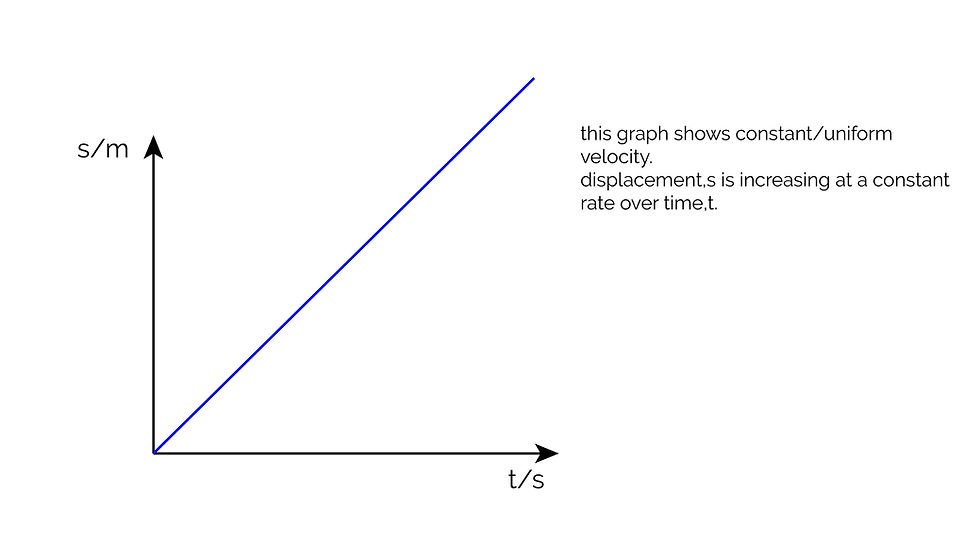cum
top of page
Search

# CSEC Physics: Motion in a Straight Line

Before we discuss the dynamics of motion, there are a few terms that you must understand:

Distance, s (a scalar quantity) is the total length of space between two points.

Displacement, s (a vector quantity) is the distance moved in a specific direction, or an object's overall change in position.Speed, v (a scalar quantity) is the distance moved per unit time.

Velocity, v (a vector quantity) is the rate at which an object moves in a certain direction, or the displacement per unit time.

v= s/t

Acceleration, a (a vector quantity) is rate at which velocity changes, or the change in velocity per unit time.

a= (v-u)/t

where v = final velocity;

u = initial velocity

t = time taken

Motion Graphs

We can plot a graph that describes the motion of an object:

Displacement-Time Graphs

These graphs have displacement on the y axis and time on the x axis. Hence, the gradient of a displacement-time graph gives the velocity of the object.Graph 1:Graph 2Graph 3Graph 4Velocity-Time Graphs

These graphs have velocity on the y axis and time on the x axis. Hence, the gradient of a velocity-time graph gives the acceleration of the object.Graph 1Graph 2Graph 3Graph 4Graph 5Knowing all of this, you should be able to describe and object's motion based on its motion graph or vice versa:If the graph above describes the motion of a truck, then:

OA- the truck is accelerating uniformly from rest for t1 seconds until it reaches a velocity of v1.

AB- the truck's acceleration decreases slightly in t2-t1 seconds from v1 to v2.

BC- the truck moves with a constant/uniform velocity of v2 for t3-t2 seconds. The truck does not accelerate.

CD- the truck decelerates uniformly from v2 to rest in t4-t3 seconds.